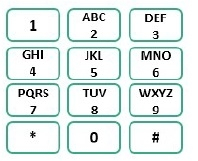In this problem, a Numeric mobile keypad is given. We can only press top, bottom, right and left buttons of the current button, diagonal keys are not Allowed. We also cannot press the * and # buttons in the keypad.A digit is given, we have to find the number of possible numbers of given digits can be formed, using the keypad, maintaining given rules.

## Input and Output

Input:
Digit count. Say 3 digit numbers.
Output:
Number of possible 3 digit numbers, that can be formed with the given conditions. Here the answer is 138.

## Algorithm

getCount(n)

Input: number of digits n.
Output: Possible ways to type n digit numbers in a mobile keypad.

Begin
if n <= 0, then
return 0
if n = 1, then
return 10

define two array row and col to move each direction from current key
define count table of size (10 x n+1)

for i in range 0 to 9, do
count[i, 0] := 0
count[i, 1] := 1
done

for k in range 2 to n, do
for i in range 0 to 3, do
for j in range 0 to 2, do
if key[i, j] ≠ * or #, then
num := key[i, j]
count[num, k] := 0
for all possible moves, do
rowMove := i + row[move]
colMove := j + col[move]
if rowMove in (0..3) colMove in (0..2), and key ≠ * or #, then
nextNum := key[rowMove, colMove]
count[num, k] := count[num, k] + count[nextNum, k+1]
done
done
done
done

totalCount := 0
for i in range 1 to 9, do
totalCount := totalCount + count[i, n]
done

End

## Example

#include <iostream>
using namespace std;

{'1','2','3'},
{'4','5','6'},
{'7','8','9'},
{'*','0','#'}
};

int getCount(int n) {
if(keypad == NULL || n <= 0)
return 0;

if(n == 1)
return 10;       //1 digit number 0-9

int row[] = {0, 0, -1, 0, 1};           //for up and down the row will change

int col[] = {0, -1, 0, 1, 0};           //for left and right column will change

int count[n+1];                    //store count for starting with i and length j
int move=0, rowMove=0, colMove=0, num = 0;
int nextNum=0, totalCount = 0;

for (int i=0; i<=9; i++) {                 //for length 0 and 1
count[i] = 0;
count[i] = 1
}

for (int k=2; k<=n; k++) {                   //for digits 2 to n
for (int i=0; i<4; i++ ) {                 //for Row wise
for (int j=0; j<3; j++) {              // for column wise
if (keypad[i][j] != '*' && keypad[i][j] != '#') {   //keys are not * and #
num = keypad[i][j] - '0';                //find the number from the character
count[num][k] = 0;

for (move=0; move<5; move++) {
rowMove = i + row[move];          //move using row moving matrix
colMove = j + col[move];          //move using column moving matrix
if (rowMove >= 0 && rowMove <= 3 && colMove >=0 && colMove <= 2 &&
nextNum = keypad[rowMove][colMove] - '0';        //find next number
count[num][k] += count[nextNum][k-1];
}
}
}
}
}
}

totalCount = 0;
for (int i=0; i<=9; i++)             //for the number starting with i
totalCount += count[i][n];
}

int main() {
int n;
cout << "Number of digits: "; cin >> n;
cout << "Possible Combinations: " << getCount(n);
}

## Output

Number of digits: 3
Possible Combinations: 138